# Loss Functions

eulerr features multiple loss functions, which result in different diagrams for many combinations. In this vignette, we visualize the effect of the loss function on an example from an issue posted on the GitHub repository for eulerr.

We list the combinations below, which consists of 5 different sets, agc, camk, cmgc, and tk.

combos <- c(
"agc"                  = 9,
"camk"                 = 17,
"cmgc"                 = 16,
"tk"                   = 16,
"tkl"                  = 23,
"agc&camk"             = 1,
"camk&tk"              = 1,
"tk&tkl"               = 1,
"camk&cmgc&tkl"        = 1,
"camk&tk&tkl"          = 2,
"agc&camk&tk&tkl"      = 1,
"camk&cmgc&tk&tkl"     = 3,
"agc&camk&cmgc&tk&tkl" = 1
)

Notice that the sizes of most of the intersections are small compared to the size of the sets themselves and that many of the intersections are missing. Generating an exact Euler diagram that shows these intersections and at the same time omits the intersections that are here implicitly 0 is an impossible problem, which means that the best we can do is an approximation.

What kind of approximation we get depends on the loss functions we use. If we use the default, which in eulerr is the sums of squared errors, we will almost certainly get a design in which the intersections involving many sets are missing since including them inevitably leads to larger errors from having to include other intersections that are not present.

library(eulerr)

fit <- euler(combos)
plot(fit)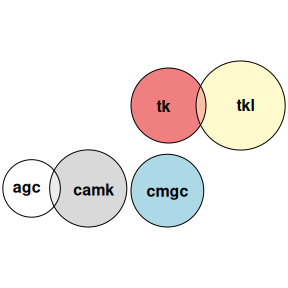An Euler diagram fit to the combination given earlier on, showing that only 1-by-1 intersections are present. This fit uses the default loss function, the sum of squared errors.

If we rather want a diagram that includes these intersections, despite leading to errors for the zero-intersections, then we need to switch the loss function we use. In eulerr, you can do so via the two arguments loss and loss_aggregator in euler(). We start by listing the alternatives for the loss argument.

Loss functions in eulerr
Loss Input Definition
Squared errors square $$(y_i - \hat y_i)^2$$
Absolute errors abs $$|y_i - \hat y_i|$$
RegionErrors region $$\big|y_i/\sum_k y_k - \hat y_i / \sum_k \hat y_k \big|$$

How the final loss is computed depends on the value of loss_aggregator, which is the function used to aggregate the values computed for each set intersection via the function used in loss. The two available settings are "sum" and "max", which should be self-explanatory.

That means that loss = "square" and loss_aggregator = "sum" leads to the sum of squared errors. loss = "region" uses regionError, which is a loss metric introduced by (Micallef and Rodgers 2014). Together with loss_aggregator = "max", euler() will use diagError (introduced in the same paper).

To see what these different choices mean for the combination that we have looked at, we now refit the diagram for each combination.

losses <- c("square", "abs", "region")
aggregators <- c("sum", "max")

for (loss in losses) {
for (aggregator in aggregators) {
fit <- euler(combos, loss = loss, loss_aggregator = aggregator)
print(plot(fit, main = paste(aggregator, loss, sep = ", ")))
}
}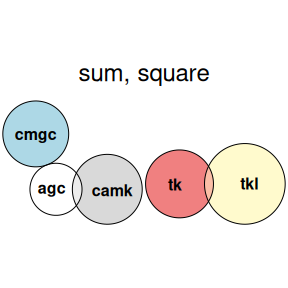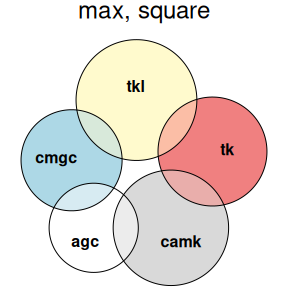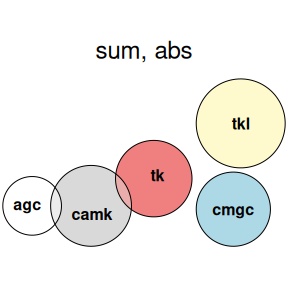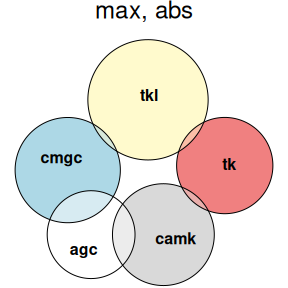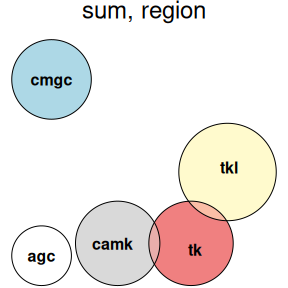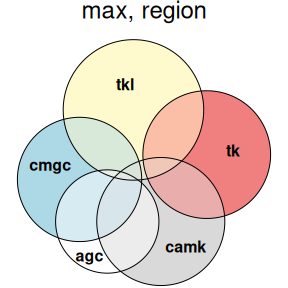As you can see, the errors that sum either the absolute or squared errors result in very similar fits and keep the existing two-set intersections and drop everything else. The abs + max and max + square combos, meanwhile, produce fits that are much more unpredictable since they only care about the largest error. Finally, diagError results in diagrams that tries to include many more intersections at the cost of reducing the goodness-of-fit of the larger intersections.

Feel free to raise a request (or better yet, a pull request) at https://github.com/jolars/eulerr/issues if you know of any other loss function that you think should be included in the package.

Micallef, Luana, and Peter Rodgers. 2014. eulerAPE: Drawing Area-Proportional 3-Venn Diagrams Using Ellipses.” PLOS ONE 9 (7): e101717. https://doi.org/10.1371/journal.pone.0101717.